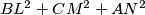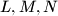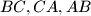### IMO Shortlist 1987 problem 5

Kvaliteta:
Avg: 0,0
Težina:
Avg: 0,0
Find, with proof, the point$P$ in the interior of an acute-angled triangle$ABC$ for which$BL^2+CM^2+AN^2$ is a minimum, where$L,M,N$ are the feet of the perpendiculars from$P$ to$BC,CA,AB$ respectively.

Proposed by United Kingdom.
Izvor: Međunarodna matematička olimpijada, shortlist 1987